# How do you use summation(∑) in LaTeX?

LaTeX provides us with the default or amsmath package which gives us commands to beautifully typeset summations.

In this article, we shall explore the various commands to typeset summations with numerous examples.

In mathematics,  summation is denoted by the Greek capital letter sigma(∑). The command for displaying a summation sign is \sum and \Sigma.

Although \Sigma and \sum return the same type of symbol.

In the case of \Sigma, size of the symbol is small but in the case of \sum, size of symbol and size of expression are adjustable.

\documentclass{article}
\begin{document}
$\Sigma x_i \qquad \sum x_i$
$\Sigma \frac{1}{x_i} \qquad \sum \frac{1}{x_i}$
$\Sigma_{i=1}^k x_i \qquad \sum_{i=1}^k x_i$
\end{document}

Output :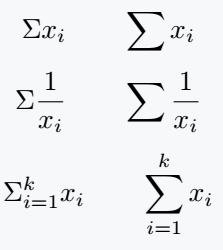## Summation with above and below limits

the general syntax for typesetting summation with above and below limits in LaTeX is \sum_{min}^{max}.

\documentclass{article}
\usepackage{amsmath}
\begin{document}
Summation in Mathematics is denoted by the symbol $\sum$ and for a set of real numbers $x_1, x_2, \cdots, x_n$, their sum is defined as $\sum_{k=1}^{n}x_k$ . That is
$\sum_{k=1}^{n}x_k = x_1 + x_2 + \cdots + x_{n-1} + x_n$
\end{document}

Output :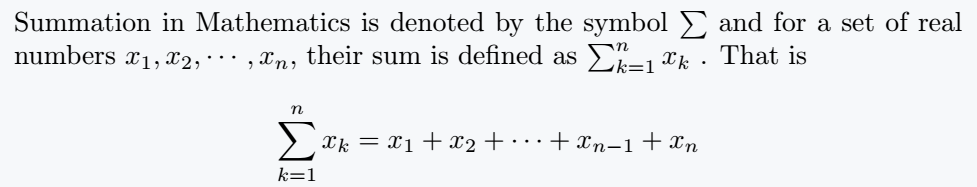Summation sign together with the limits appears differently in the inline mode and display mode.

In inline mode limits appear next to the symbol and in the display mode the limit appear below and above the summation sign.

The are two commands that are capable of controlling how these limits appear.

First is the \limits command which forcefully pushes the limits below and above the summation sign in all the math modes and the second is the \nolimits command which reverse the effect(forces the limits to stay next to the summation symbol in all math modes).

\documentclass{article}
\usepackage{amsmath}
\begin{document}
\begin{center}
\hline
\multicolumn{2}{|c|}{Summation in math mode} \\
\hline
inline math mode & display math mode \\
$\sum_{k=1}^n$ & $\sum_{k=1}^n}$\\
\hline
\multicolumn{2}{|c|}{Summation in math mode with \color{teal}{\textbackslash{}limits}}\\
\hline
$\sum\limits_{k=1}^n$ & $\sum\limits_{k=1}^{n}}$\\
\hline
\multicolumn{2}{|c|}{Summation in math mode with \color{teal}{\textbackslash{}nolimits} }\\
\hline
$\sum\nolimits_{k=1}^n$ & $\sum\nolimits_{k=1}^{n}$\\
\hline
\end{tabular}
\end{center}
\end{document}## Double and triple sums

You can easily typeset double and triple integrals by using the sum(summation) LaTeX command twice and trice. It follows for multiple sums.

\sum\sum = ∑ ∑ and \sum\sum\sum = ∑ ∑ ∑

\documentclass{article}
\begin{document}
$\sum\limits_{i=1}^m\sum\limits_{j=1}^n x_i y_j$
$\mathrm{Find} \sum\limits_{i=1}^\infty\sum\limits_{j=1}^\infty\sum\limits_{k=1}^\infty \frac{1}{a^{i+j+k}}=?\;\mathit{if}|\mathit{a}|>1$
\end{document}

Output :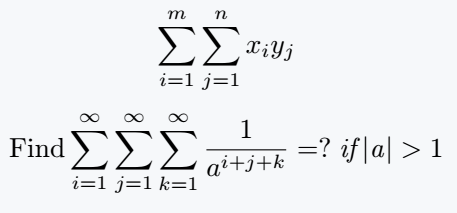## Use two and multiple subscripts under Sum

1. \atop : This command permit you to write two lines under your sum notation. For example

\documentclass{article}
\begin{document}
$\sum_{ 1 \leq i \leq n \atop 1 \leq j \leq n }x_{i,j}$
\end{document}

Output :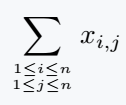2. \substack{argument} : This command permit you to write multiple lines under the sum notation by taking the lines as arguments and breaking them using the newline command \\. For example

\documentclass{article}
\usepackage{amsmath}
\begin{document}
$\sum_{\substack{ 1 \leq i \leq n \\ 1 \leq j \leq n \\ 1 \leq i \leq j \leq n\\ \cdots }}$
\end{document}

Output :3. \begin{subarray}{column_definition} \cdots \end{subarray}: This environment permits you to achieve the same effect as \substack{argument}. For example:

\documentclass{article}
\usepackage{amsmath}
\begin{document}
$\sum_{\begin{subarray}{c} 1 \leq i \leq n \\ 1 \leq j \leq n \\ 1 \leq i \leq j \leq n\\ \cdots \\ \end{subarray} }$
\end{document}

Output :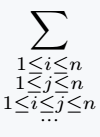4. \sideset{left}{right}{symbol} makes it possible for you to place a number or a symbol at all four corners of the sum symbol.

It equally permit you place characters which will not be possible to place them using limits for example putting prime in-front of the sum symbol in the desired position is only possible with this command. For example:

\documentclass{article}
\usepackage{amsmath}
\begin{document}
$\sideset{_{\alpha} ^{\beta}} {_{\gamma} ^{\sigma}}\sum_{ 1 \leq i \leq n \atop 1 \leq j \leq n }x_{i,j}$
adding a prime to the sum
$\sideset{}{'}\sum_{ 1 \leq i \leq n \atop 1 \leq j \leq n }x_{i,j}$
\end{document}

Output :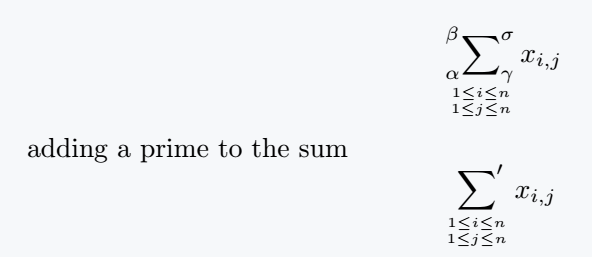## Some examples

As you can easily understand, the sum symbol is used in the following different types of mathematical expressions.

\documentclass{article}
\usepackage{amssymb}
\begin{document}
or all $n \in \mathbb{N}^{\ast}$ , we have
$\sum_{k=1}^{n}k = \frac{n(n+1)}{2}, \quad \sum_{k=1}^{n}k^2 = \frac{n(n+1)(2n+1)}{6}, \quad \sum_{k=1}^{n}k^3 = \left(\frac{n(n+1)}{2}\right)^2$
\end{document}

Output :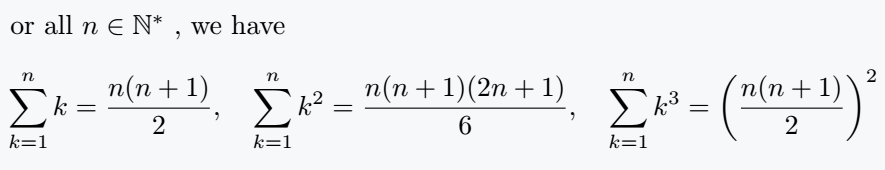Geometric sum

\documentclass{article}
\usepackage{amssymb,amsmath}
\begin{document}
$\forall n \in \mathbb{N}, \quad \sum\limits_{k=0}^{n}q^k =\begin{cases} n + 1, & \text{if$ q = 1 $}\\ \frac{1-q^{n+1}}{1-q}, & \text{if$ q \neq 1 $} \end{cases}$
\end{document}

Output :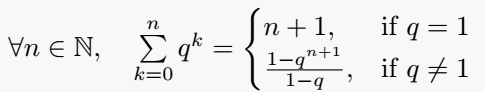\documentclass{article}
\usepackage{amsmath}
\begin{document}
Interchanging double sums when the indices are dependent
$\sum_{1 \leq i \leq j \leq n}x_{i,j} = \sum_{i = 1}^{n}\sum_{j=1}^{n}x_{i,j} = \sum_{ j = 1}^{n}\sum_{i=1}^{j}x_{i,j} \; \text{and}\; \sum_{1 \leq i \leq j \leq n}x_{i,j} = \sum_{i=1}^{n-1}\sum_{j = i+1}x_{i,j} = \sum_{j=2}^{n}\sum_{i=1}^{j-1}x_{i,j}$
Commutativity and associativity property of double sums
$\sum_{k \leq j \leq i \leq n}a_{ij} = \sum_{i=k}^{n}\sum_{j=k}^{i}a_{i,j} = \sum_{j=k}^{n}\sum_{i=j}^{n}a_{ij} = \sum_{j=0}^{n-k}\sum_{i=k}^{n-j}a_{i+j,i}$
\end{document}

Output :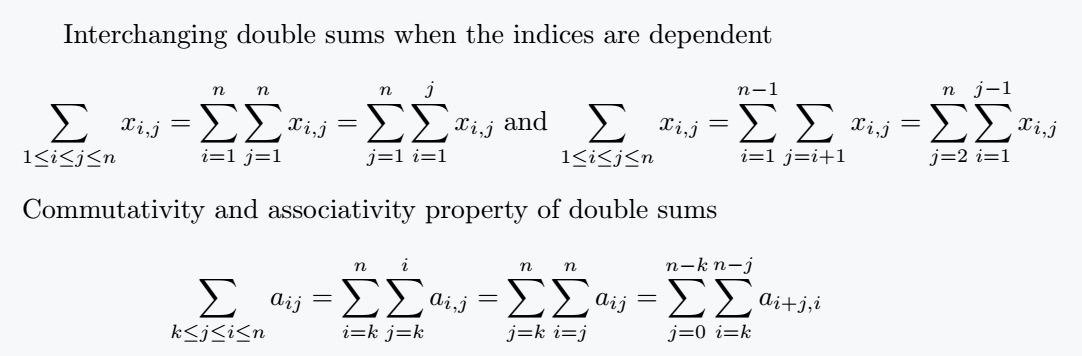## Conclusion

This article gives you a full coverage of how to typeset summations in LaTeX and some few related commands.

A good mastery of this tutorial will improve your mathematical typesetting and make you an efficient typesetter of higher mathematics.#### Md Jidan Mondal

LaTeX expert with over 10 years of experience in document preparation and typesetting. Specializes in creating professional documents, reports, and presentations using LaTeX.